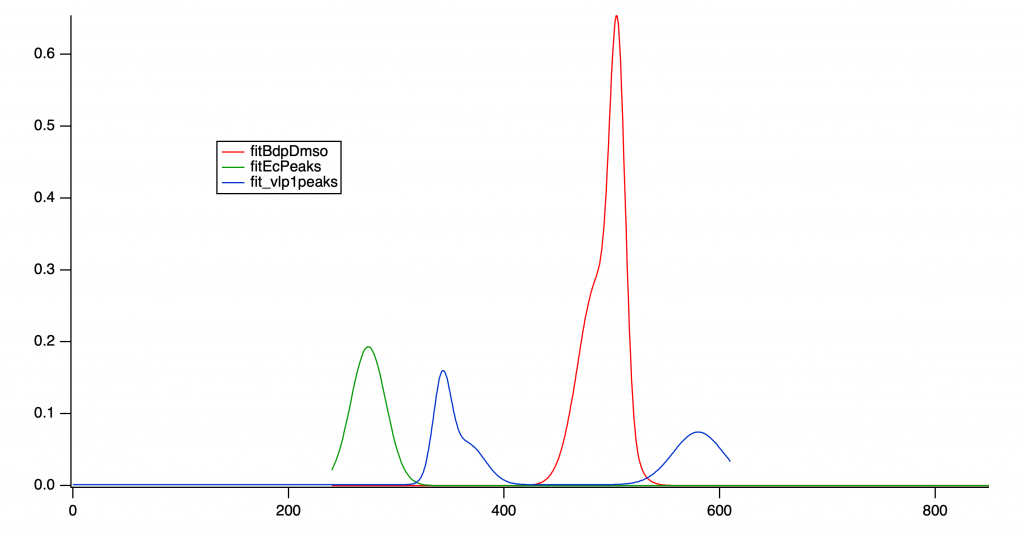# Linear combination of two waves?Hello! Pretty new to Igor here–but this is essentially what I'm trying to do:

- Take two waves (A and B) and find a linear combination of them such that they fit another wave (C)

- Determine the coefficients that make the correct linear combination of A and B

I'm having trouble finding the coefficients–I tried using MatrixLLS but don't really know how to use it. Do A, B, and C all have to be of the same dimension?

I've included my data file below (apologies for it being so messy!)–mostly take a look at Graph2. I'm trying to find linear combinations of fit_ecpeaks and fit_bdpdmso to fit fit_vlp1peaks, fit_vlp2peaks, and fit_vlp3peaks. Any help is greatly appreciated!

Hi,

The first path I looked to your request was a simple linear combination using 2D polynomial fit but I could not proceed because as you mentioned the waves are different length.

Fit_vlp1Peaks is 611points

fitBdpDmso is 595 points

fitEcPeaks is 311 points

and a quick graph does reveal a linear combination seems likely.Is there a bit more to your request?

Andy

In reply to by hegedus

Do you have any suggestions for how to go about finding the linear combination? I tried concatenating fit_bdpdmso with fit_ecpeaks, then using MatrixLLS:

``````Concatenate {fit_bdpdmso, fit_ecpeaks}, ecAndBdp
MatrixLLS ecAndBdp fit_vlp1peaks
print m_B, m_B``````

But the output of the last statement was just 0 0, which are supposedly the coefficients for the LC.

You can do this with curve fitting.

The waves on the plot are buried within a package datafolder. First go to the data browser and set the data folder to that of your fit and reference waves. Better still, make a copy of the waves in your working data folder, rather than trying to work in a folder that's reserved for a package.

Then open the curve fitting dialog and create a new fit function.

the independent variable is x, and you need two coefficients a and b.

in the body of the function type this:

wave ec = fit_ecpeaks, bd = fit_bdpdmso
f(x) = a * ec(x) + b * bd(x)

then return to the curve fitting dialog, set initial guesses for a and b, and you're ready to fit.